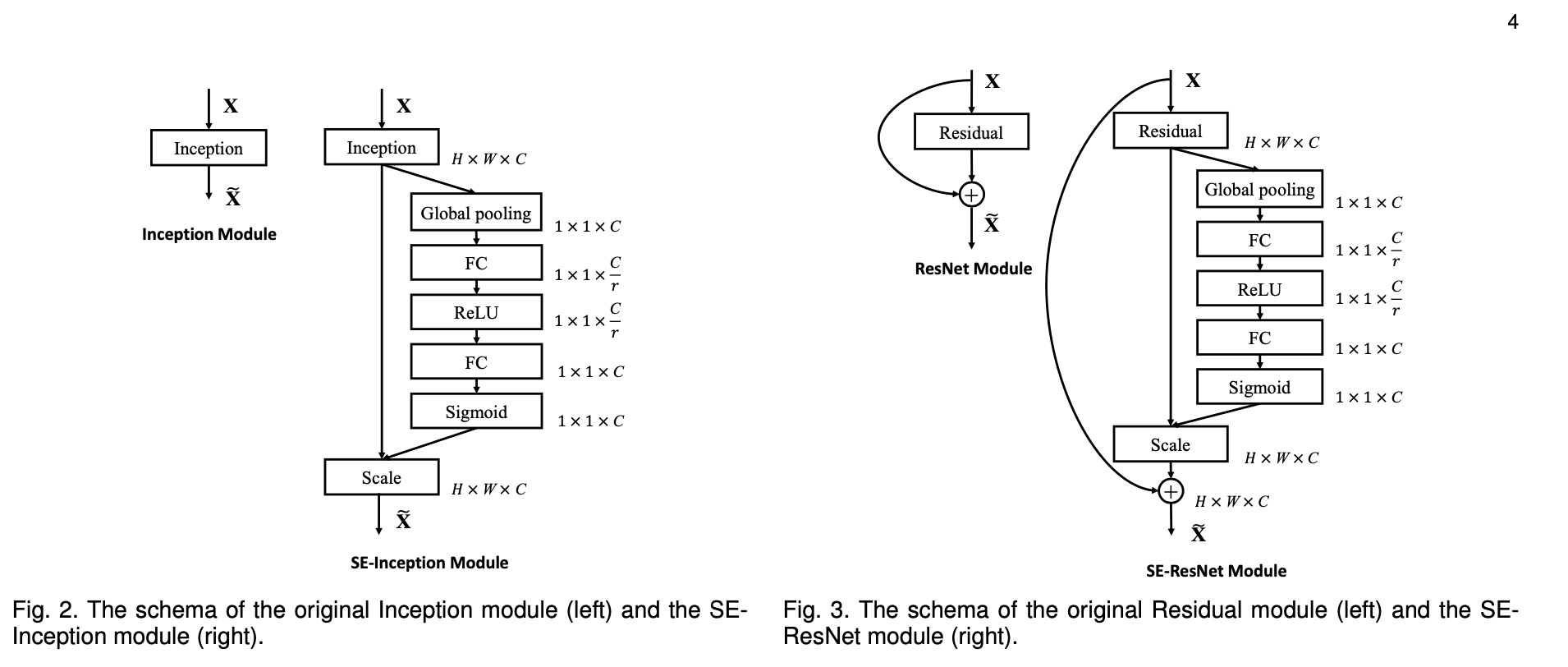# Squeeze-and-Excitation Networks

Squeeze-and-Excitation Networks（SENet)是CVPR2018公布的一种全新的图像识别结构，它通过对特征通道间的相关性进行建模，把重要的特征进行强化来提升准确率。是目前流行的一种子结构

## SQUEEZE-AND-EXCITATION BLOCKS### Squeeze: Global Information Embedding

$$z_c = F_s(U_c) = \frac{1}{H ✖️ W}\sum^H_i\sum^W_j u_c(i,j)$$

• 能够学得各个 channel 之间的非线性相互关系
• 必须学的是非互斥得关系，因为可以有多个 channel 被强调，而不是 one-hot 激活

$$s = F_e(z, W) = \sigma(g(z, W)) = \sigma(W_2\delta(W_1z))$$

$W_1$, $W_2$为全连接， $\delta$ 为 relu 激活. 对于激励模块，作者首先使用一个全连接层对 GAP 结果以 $\frac{C}{r}$降维, 然后接 relu 激活函数学习非线性相关性，之后再跟一个全连接恢复 channel 维度.### usage

• SE-block 可用于分类与检测. 对于标准网络结构如VGGNet，SE-block 可以用在每一个卷积+非线性操作之后. $Fig.2$和$Fig.3$分别是SE-Inception module和SE-Resnet module.
• ratio. 在ImageNet上，作者得到的最佳ratio为16,可做相对参考

### 简单代码(keras)


def squeeze_excitation_layer(x, ratio, out_dim):

squeeze = GlobalAveragePooling2D()(x)
excitation = Dense(units=out_dim // ratio)(squeeze)
excitation = Activation('relu')(excitation)
excitation = Dense(units=out_dim)(excitation)
excitation = Activation('sigmoid')(excitation)
excitation = Reshape((1, 1, out_dim))(excitation)
scale = multiply([x, excitation])

return scale